Next: Clique Graphsk-null Ordered Up: Applications/New Directions Previous: The Cancellation Problem

## Results on the Structure of Fixed Point Sets

Aside from the determination whether an ordered set has the fixed point property it certainly is interesting to determine the structure of the fixed point sets. The earliest result in that direction is Theorem 1 in  (the fixed point sets of order-preserving maps of a complete lattice are complete lattices themselves) and another well-known result is Theorem 3 in  (in finite dismantlable ordered sets the fixed point sets of order-preserving mappings are dismantlable). Surprisingly, despite the apparent strength of the fixed point property, there are ordered sets with the fixed point property that have an order-preserving map whose set of fixed points is disconnected. Examples are the set P2 in  (here also depicted in Figure 3 as the set P) and the set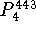in . The following is a sufficient criterion for the fixed point sets to be connected.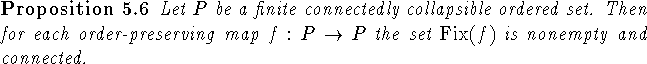Proof: Nonemptyness of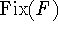follows for example from Theorem 4.27 or directly from Theorem 3.4 via an easy induction. The proof of connectedness is an induction on n:=|P|. For n=1 there is nothing to prove. For the step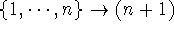let P be an (n+1)-element connectedly collapsible ordered set and let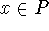be as in the definition of connected collapsibility. Let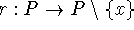be a retraction and let b:=r(x). By definition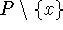and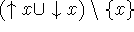are connectedly collapsible and clearly both sets have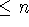elements. Thus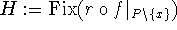is connected. Clearly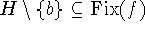, and thus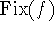must be one of the following four sets: H,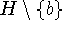,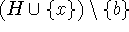,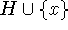. The case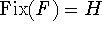is trivial. In all the other cases we have to show that any two elements ofare joined by a fence in.
In case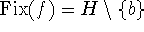we are trivially done if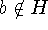, so we will assume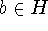. Since r(f(b))=b and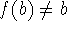we infer f(b)=x. Moreover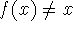. Let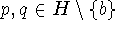and let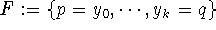be a fence in H. If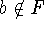, then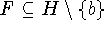and we are done. If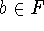, we can assume without loss of generality that there is exactly one index m such that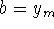and we can assume that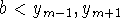. If f(x) is not related to x, then (since f(b)=x) f mapsto itself and thus sinceis connectedly collapsible withelements,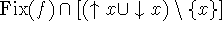is connected. Hence there is a fence from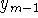to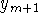that lies entirely inand thus there is a fence from p to q in. If f(x) is related to x, there is a smallest fixed point of f that is above x or a largest fixed point of f that is below x. Call this fixed point c. Then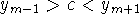and p and q are joined by a fence in.
In case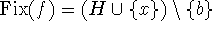again we will assume that(the caseis treated when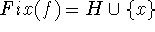). Again we infer f(b)=x. If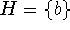, then we must have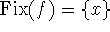and we are done. Otherwise there is a fixed point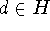of f that is related to b and not equal to b or x. Since f(b)=x, we have that d is related to x also. Let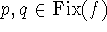. Since d is related to x we can assume that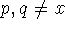. Letbe a fence in H. Again we are done unlessfor exactly one m and (without loss of generality). Since f(b)=x, we have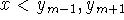and p and q are joined by a fence in.
Finally in case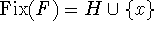let us first assume. Then we are done if x is comparable to b. Otherwise f mapsto itself, so x and b have a common upper bound inand thusis connected. In case, we have that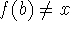. If f(b) is related to x (and thus to b), then there is a point in H that is above x or below x and thusis connected. If f(b) is not related to x, then f mapsto itself. Hence again there is a point in H that is above x or below x and thusis connected. \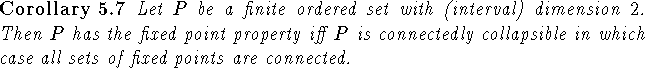Proof: It is proved by Fofanova, Rival and Rutkowski in  that every ordered set of dimension 2 has a retractable point. The same result is proved for interval dimension 2 in . Since (interval) dimension is a hereditary concept this means that each ordered set of (interval) dimension 2 is collapsible. The result now follows from Theorem 4.27 and Proposition 5.6. \Next: Clique Graphsk-null Ordered Up: Applications/New Directions Previous: The Cancellation Problem

Bernd.S.W.Schroder